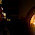Tuesday, January 25, 2011

Upcoming Workshop: Constructive Mathematics

"What constructive mathematicians actually do"
University of Melbourne

Maarten McKubre-Jordens, University of Canterbury, New Zealand

I) Introduction to constructive mathematics
11:00am, Monday 14 February 2011, Old Arts-227 (Cecil Scutt Collaborative Teaching Room)

In the first of this two-part workshop, constructive mathematics is characterised as reasoning from positive definitions.
Several different schools (or models) of constructive mathematics are outlined, together with a common base. Some important non-constructive principles are outlined and and their impact evaluated, from the point of view of the practising mathematician. A small amount of familiarity with classical logic and mathematics will be assumed.

II) Constructive mathematics in action

11:00am, Wednesday 16 February 2011, Old Arts-227 (Cecil Scutt Collaborative Teaching Room)

What is the constructive role of examples, and counterexamples, in mathematics? What are some common techniques for proving theorems constructively? The second part of this workshop surveys how the role of examples in mathematical thought sets apart constructive thought, and will briefly discuss some typical strategies for proving theorems. Time permitting, we will investigate the idea of reverse mathematics as practised constructively.

III) Strange encounters and the importance of constructive thought

11:00am, Friday 18 February 2011, Old Quad Moot Court

Everyone has heard of some variation of the infinite monkey theorem:

Theorem. In an infinite collection of monkeys, given typewriters and a suitable amount of time, there is a monkey that with probability 1 reproduces the entire collected works of Shakespeare.

Of course this is not rigorously worded, but you get the idea. Now, how do you find the cheeky monkey that actually did it? In this seminar we take a look at some of the stranger and more intriguing aspects of constructive analysis — such as strictly increasing bounded sequences that do not converge, and well-defined sets in R^n from which we cannot calculate distances — and discuss their impact.

1 comment:

1.Thanks for a great week, Maarten!